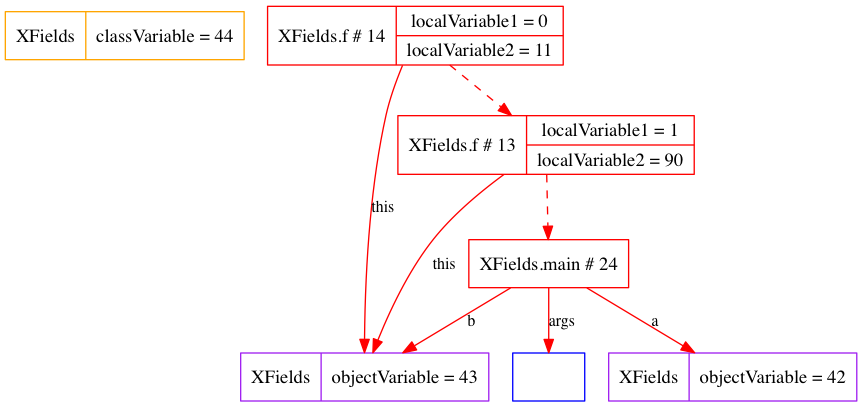# CSC301: Week 3: Symbol Tables (3.1, 3.5)

 Contents [0/8]

 Homework [1/8] Storage: Variables and fields [2/8] Storage: Is this correct? [3/8] Storage: Is this correct? [4/8] Storage: Is this correct? [5/8] Storage: Is this correct? [6/8] Storage: Is this correct? [7/8] Storage: Is this correct? [8/8]

 Homework [1/8]

• Read Algorithms 3.1, 3.5 and 3.2

• Do the following problems from the text. (Not handed in.)

```  3.1.10

Give a trace of the process of inserting the keys
E A S Y Q U E S T I O N
into an initially empty table using SequentialSearchST. How many
compares (of keys) are involved?

3.1.11

Give a trace of the process of inserting the keys
E A S Y Q U E S T I O N
into an initially empty table using BinarySearchST. How many
compares (of keys) are involved?
```

 Storage: Variables and fields [2/8]

Storage

• Local variable (including method parameters): stored with record for each method call. (In reality, determined by compiler. The compiler freely introduces and removes local variables. They may be stored in register or not stored at all.)
• Object variable (field): stored with object.
• Class variable (static field): stored with class.

Sharing

• Local variable: separate for each method call, even on the same object.
• Object variable (field): shared by method calls on the same object.
• Class variable (static field): shared by all objects.

Initialization

• Local variable: initialized when method called.
• Object variable (field): initialized when object is first created.
• Class variable (static field): initialized when class is loaded (before any object created).

 file:XFields.java [source] [doc-public] [doc-private]
 ``` 01 02 03 04 05 06 07 08 09 10 11 12 13 14 15 16 17 18 19 20 21 22 23 24 25 26 27 ``` ```package algs12; import stdlib.*; public class XFields { public static int classVariable = 42; public int objectVariable = classVariable++; public void f(int localVariable1) { int localVariable2 = StdRandom.uniform (100); if (localVariable1 > 0) f (localVariable1 - 1); } public static void main (String[] args) { Trace.drawSteps (); Trace.run (); XFields a = new XFields (); XFields b = new XFields (); a.f(1); b.f(1); } } ```

Here is the state just before the main function ends:(This example revealed a bug in my trace software. You can download a corrected copy here: file:Trace.java)

 Storage: Is this correct? [3/8]

```    public int size () {
return size (root, 0);
}
private static int size (Node x, int sz) {
if (x == null) return sz;
sz = sz + 1;
size (x.left, sz);
size (x.right, sz);
return sz;
}```

Recall this broken version of `size`

Mistake here is to think of `sz` as shared among the function calls.

 Storage: Is this correct? [4/8]

```    public int size () {
return size (root);
}
private static int sz = 0;
private static int size (Node x) {
if (x == null) return sz;
sz = sz + 1;
size (x.left);
size (x.right);
return sz;
}```

Is this correct?

 Storage: Is this correct? [5/8]

```    public int size () {
return size (root);
}
private static int sz = 0;
private static int size (Node x) {
if (x == null) return sz;
sz = sz + 1;
size (x.left);
size (x.right);
return sz;
}```

Is this correct?

Only if you call size once per object. If called twice, it gives the wrong answer.

 Storage: Is this correct? [6/8]

```    public int size () {
return size (root);
}
private int sz = 0;
private int size (Node x) {
if (x == null) return sz;
sz = sz + 1;
size (x.left);
size (x.right);
return sz;
}```

A variation without `static`. Still wrong for the same reason.

 Storage: Is this correct? [7/8]

```    public int size () {
sz = 0;
return size (root);
}
private static int sz;
private static int size (Node x) {
if (x == null) return sz;
sz = sz + 1;
size (x.left);
size (x.right);
return sz;
}```

Is this correct?

 Storage: Is this correct? [8/8]

```    public int size () {
sz = 0;
return size (root);
}
private static int sz;
private static int size (Node x) {
if (x == null) return sz;
sz = sz + 1;
size (x.left);
size (x.right);
return sz;
}```

Is this correct?

Only if your code is single threaded. If two threads call size on the same object at the same time, the `sz` field will become a mess.

Revised: 2008/03/17 13:01